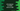# 2 ways in C# to check odd even numbers## How to check if a number is even or odd in C#:

In this tutorial, we will learn how to check if a number is even or odd using `C#`. The program will take the number as input from the user and print out the result i.e. it will print if the number is even or odd.

With this example program, you will learn:

• How to print anything on the console
• How to read user inputs in C#
• How to use if-else conditions, and
• How to use the modulo operator, `%`, in `C#`.

### `%` Operator or modulo operator in C#:

The modulo operator(`%`) computes the remainder of a division operation. `a%b` will return the remainder after dividing `a` by `b`. For example:

``````Console.WriteLine(5 % 4);
Console.WriteLine(8 % 4);
Console.WriteLine(10 % 4);
Console.WriteLine(10 % -9);
Console.WriteLine(-10 % 9);
Console.WriteLine(-10 % -9);``````

If you run the above code, it will print:

``````1
0
2
1
-1
-1``````

As you can see, it returns the remainder of the division operations of the first and the second operands. The sign of the result is the same as the first operand.

The above example used only integers but we can also use the `%` operator with floating-point numbers as well. For floating-point numbers, the result will be a float value.

### Method 1: How to find if a number is even or odd by using the modulo operator:

A number is called an even number if it is divisible by 2. Otherwise, it is called an odd number.  We will use the `%` operator to find out if a number is even or odd.

For a number `n`, `n%2` is `0` if it is an even number. If `n` is odd, it will be `1`.

#### C# Program to check if a number is even or odd:

The following `C#` program checks if a user-entered number is even or odd:

``````using System;

namespace dotnet_sample
{
class Program
{
static void Main(string[] args)
{
//1
int n;

//2
Console.WriteLine("Enter a number to check:");

//3

//4
if(n % 2 == 0)
{
//5
Console.WriteLine(n + " is an even number");
}
else
{
//6
Console.WriteLine(n + " is an odd number");
}
}
}
}``````

#### Explanation:

The commented numbers in the above program denote the step numbers below:

1. Create one integer variable `n` to assign the user-input number.

2. Ask the user to enter a number.

3. Read the number and assign it to the variable `n`.

4. With an `if-else` statement, check if the number is divisible by 2 or not.

5. If yes, print that the number is an even number.

6. Else, print that the number is an odd number.

#### Sample Output:

It will print output as below:

``````Enter a number to check :
10
10 is an even number

Enter a number to check :
12
12 is an even number

Enter a number to check :
13
13 is an odd number

Enter a number to check :
12543
12543 is an odd number``````

### Method 2: By using Bitwise `AND` operation:

The Bitwise `AND` operation of a number with 1 is 0 for a number if the number is even. It will be 1 if the number is odd. The symbol of Bitwise `AND` operation is `&`. For a number `n`, the result of `n & 1` is 0 if `n` is odd. Else, it is even.

The following program shows how to use the Bitwise `AND` operation to check if a number is odd or even:

``````namespace dotnet_sample
{
class SecondExample
{
static void Main(string[] args)
{
int n;

Console.WriteLine("Enter a number to check:");

if ((n & 1) == 0)
{
Console.WriteLine(n + " is an even number");
}
else
{
Console.WriteLine(n + " is an odd number");
}
}
}
}``````

It uses the Bitwise `AND` operation with 1 to check if the number is even or not. If the statement `(n & 1) == 0` is `True`, the integer `n` will be an even number. Else, it will be an odd number.
We have learned how to check if a number is even or not in `C#` in two ways. Please raise a pull request on GitHub if you want to add anything to the programs.